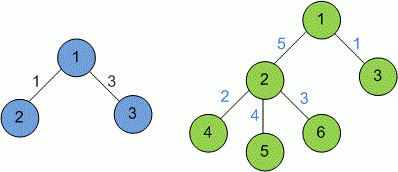Competitions

# Sum

Roman’s parents gave him an undirected connected weighted graph with n vertexes and n - 1 edges. Roman wants to know the sum of all the paths’ lengths in this graph. Let’s consider path’s length as sum of all the edges that lay on this path. Roman said that the path from u to v is the same as from v to u, so he doesn’t distinguish them.

#### Input

The first line contains the single integer number n (2n105) – the number of vertexes in graph. It is followed by n - 1 lines containing the description of the edges. Every line of description consists of three integer numbers: vertices connected by the edge (labeled from 1 to n) and edge’s weight.

#### Output

Print he sum of all the paths’ lengths in the given graph modulo 109.Time limit 1 second
Memory limit 128 MiB
Input example #1
3
1 2 1
1 3 3

Output example #1
8

Input example #2
6
1 2 5
1 3 1
2 4 2
2 5 4
2 6 3

Output example #2
90


Example description: An explanation to the example: All the paths are 1->2, 1->3, 2->1->3 and their lengths’ sum is 1+3+4=8.

Source 2011 International Collegiate Programming Contest, Ukraine, Quarter-Final, May 19